• ## 算法学习之哈夫曼编码算法

万次阅读 多人点赞 2017-04-04 12:16:03
哈夫曼编码算法用字符在文件中出现的频率表来建立一个用0，1串表示各字符的最优表示方式。一个包含100,000个字符的文件，各字符出现频率不同，如下表所示。  有多种方式表示文件中的信息，若用0,1码表示...
     1、问题描述
哈夫曼编码是广泛地用于数据文件压缩的十分有效的编码方法。其压缩率通常在20%～90%之间。哈夫曼编码算法用字符在文件中出现的频率表来建立一个用0，1串表示各字符的最优表示方式。一个包含100,000个字符的文件，各字符出现频率不同，如下表所示。有多种方式表示文件中的信息，若用0,1码表示字符的方法，即每个字符用唯一的一个0,1串表示。若采用定长编码表示，则需要3位表示一个字符，整个文件编码需要300,000位；若采用变长编码表示，给频率高的字符较短的编码；频率低的字符较长的编码，达到整体编码减少的目的，则整个文件编码需要（45×1+13×3+12×3+16×3+9×4+5×4）×1000=224,000位，由此可见，变长码比定长码方案好，总码长减小约25%。
前缀码：对每一个字符规定一个0,1串作为其代码，并要求任一字符的代码都不是其他字符代码的前缀。这种编码称为前缀码。编码的前缀性质可以使译码方法非常简单；例如001011101可以唯一的分解为0,0,101,1101，因而其译码为aabe。

译码过程需要方便的取出编码的前缀，因此需要表示前缀码的合适的数据结构。为此，可以用二叉树作为前缀码的数据结构：树叶表示给定字符；从树根到树叶的路径当作该字符的前缀码；代码中每一位的0或1分别作为指示某节点到左儿子或右儿子的“路标”。从上图可以看出，表示最优前缀码的二叉树总是一棵完全二叉树，即树中任意节点都有2个儿子。图a表示定长编码方案不是最优的，其编码的二叉树不是一棵完全二叉树。在一般情况下，若C是编码字符集，表示其最优前缀码的二叉树中恰有|C|个叶子。每个叶子对应于字符集中的一个字符，该二叉树有|C|-1个内部节点。
给定编码字符集C及频率分布f,即C中任一字符c以频率f(c)在数据文件中出现。C的一个前缀码编码方案对应于一棵二叉树T。字符c在树T中的深度记为dT(c)。dT(c)也是字符c的前缀码长。则平均码长定义为：使平均码长达到最小的前缀码编码方案称为C的最优前缀码。

2、构造哈弗曼编码
哈夫曼提出构造最优前缀码的贪心算法，由此产生的编码方案称为哈夫曼编码。其构造步骤如下：
(1)哈夫曼算法以自底向上的方式构造表示最优前缀码的二叉树T。
(2)算法以|C|个叶结点开始，执行|C|－1次的“合并”运算后产生最终所要求的树T。
(3)假设编码字符集中每一字符c的频率是f(c)。以f为键值的优先队列Q用在贪心选择时有效地确定算法当前要合并的2棵具有最小频率的树。一旦2棵具有最小频率的树合并后，产生一棵新的树，其频率为合并的2棵树的频率之和，并将新树插入优先队列Q。经过n－1次的合并后，优先队列中只剩下一棵树，即所要求的树T。
构造过程如图所示：具体代码实现如下：
(1)4d4.cpp，程序主文件

[cpp]
view plain
copy

//4d4 贪心算法 哈夫曼算法   #include "stdafx.h"   #include "BinaryTree.h"   #include "MinHeap.h"   #include <iostream>    using namespace std;       const int N = 6;      template<class Type> class Huffman;      template<class Type>    BinaryTree<int> HuffmanTree(Type f[],int n);      template<class Type>    class Huffman   {       friend BinaryTree<int> HuffmanTree(Type[],int);       public:           operator Type() const            {               return weight;           }       //private:           BinaryTree<int> tree;           Type weight;   };      int main()   {       char c[] = {'0','a','b','c','d','e','f'};       int f[] = {0,45,13,12,16,9,5};//下标从1开始       BinaryTree<int> t = HuffmanTree(f,N);          cout<<"各字符出现的对应频率分别为："<<endl;       for(int i=1; i<=N; i++)       {           cout<<c[i]<<":"<<f[i]<<" ";       }       cout<<endl;          cout<<"生成二叉树的前序遍历结果为："<<endl;       t.Pre_Order();       cout<<endl;          cout<<"生成二叉树的中序遍历结果为："<<endl;       t.In_Order();       cout<<endl;          t.DestroyTree();       return 0;   }      template<class Type>   BinaryTree<int> HuffmanTree(Type f[],int n)   {       //生成单节点树       Huffman<Type> *w = new Huffman<Type>[n+1];       BinaryTree<int> z,zero;          for(int i=1; i<=n; i++)       {           z.MakeTree(i,zero,zero);           w[i].weight = f[i];           w[i].tree = z;       }          //建优先队列       MinHeap<Huffman<Type>> Q(n);       for(int i=1; i<=n; i++) Q.Insert(w[i]);          //反复合并最小频率树       Huffman<Type> x,y;       for(int i=1; i<n; i++)       {           x = Q.RemoveMin();           y = Q.RemoveMin();           z.MakeTree(0,x.tree,y.tree);           x.weight += y.weight;           x.tree = z;           Q.Insert(x);       }          x = Q.RemoveMin();          delete[] w;          return x.tree;   }

(2)BinaryTree.h 二叉树实现

[cpp]
view plain
copy

#include<iostream>   using namespace std;      template<class T>   struct BTNode   {       T data;       BTNode<T> *lChild,*rChild;          BTNode()       {           lChild=rChild=NULL;       }          BTNode(const T &val,BTNode<T> *Childl=NULL,BTNode<T> *Childr=NULL)       {           data=val;           lChild=Childl;           rChild=Childr;       }          BTNode<T>* CopyTree()       {           BTNode<T> *nl,*nr,*nn;              if(&data==NULL)           return NULL;              nl=lChild->CopyTree();           nr=rChild->CopyTree();              nn=new BTNode<T>(data,nl,nr);           return nn;       }   };         template<class T>   class BinaryTree   {       public:           BTNode<T> *root;           BinaryTree();           ~BinaryTree();              void Pre_Order();           void In_Order();           void Post_Order();              int TreeHeight()const;           int TreeNodeCount()const;              void DestroyTree();           void MakeTree(T pData,BinaryTree<T> leftTree,BinaryTree<T> rightTree);           void Change(BTNode<T> *r);          private:           void Destroy(BTNode<T> *&r);           void PreOrder(BTNode<T> *r);           void InOrder(BTNode<T> *r);           void PostOrder(BTNode<T> *r);              int Height(const BTNode<T> *r)const;           int NodeCount(const BTNode<T> *r)const;   };      template<class T>   BinaryTree<T>::BinaryTree()   {       root=NULL;   }      template<class T>   BinaryTree<T>::~BinaryTree()   {          }      template<class T>   void BinaryTree<T>::Pre_Order()   {       PreOrder(root);   }      template<class T>   void BinaryTree<T>::In_Order()   {       InOrder(root);   }      template<class T>   void BinaryTree<T>::Post_Order()   {       PostOrder(root);   }      template<class T>   int BinaryTree<T>::TreeHeight()const   {       return Height(root);   }      template<class T>   int BinaryTree<T>::TreeNodeCount()const   {       return NodeCount(root);   }      template<class T>   void BinaryTree<T>::DestroyTree()   {       Destroy(root);   }      template<class T>   void BinaryTree<T>::PreOrder(BTNode<T> *r)   {       if(r!=NULL)       {           cout<<r->data<<' ';           PreOrder(r->lChild);           PreOrder(r->rChild);       }   }      template<class T>   void BinaryTree<T>::InOrder(BTNode<T> *r)   {       if(r!=NULL)       {           InOrder(r->lChild);           cout<<r->data<<' ';           InOrder(r->rChild);       }   }      template<class T>   void BinaryTree<T>::PostOrder(BTNode<T> *r)   {       if(r!=NULL)       {           PostOrder(r->lChild);           PostOrder(r->rChild);           cout<<r->data<<' ';       }   }      template<class T>   int BinaryTree<T>::NodeCount(const BTNode<T> *r)const   {

if(r==NULL)           return 0;       else           return 1+NodeCount(r->lChild)+NodeCount(r->rChild);   }      template<class T>   int BinaryTree<T>::Height(const BTNode<T> *r)const   {       if(r==NULL)           return 0;       else       {           int lh,rh;           lh=Height(r->lChild);           rh=Height(r->rChild);           return 1+(lh>rh?lh:rh);       }   }      template<class T>   void BinaryTree<T>::Destroy(BTNode<T> *&r)   {       if(r!=NULL)       {           Destroy(r->lChild);           Destroy(r->rChild);           delete r;           r=NULL;       }   }      template<class T>   void BinaryTree<T>::Change(BTNode<T> *r)//将二叉树bt所有结点的左右子树交换   {       BTNode<T> *p;       if(r){            p=r->lChild;           r->lChild=r->rChild;           r->rChild=p; //左右子女交换           Change(r->lChild);  //交换左子树上所有结点的左右子树           Change(r->rChild);  //交换右子树上所有结点的左右子树       }   }      template<class T>   void BinaryTree<T>::MakeTree(T pData,BinaryTree<T> leftTree,BinaryTree<T> rightTree)   {       root = new BTNode<T>();       root->data = pData;       root->lChild = leftTree.root;       root->rChild = rightTree.root;   }

(3)MinHeap.h 最小堆实现

[cpp]
view plain
copy

#include <iostream>   using namespace std;   template<class T>   class MinHeap   {       private:           T *heap; //元素数组，0号位置也储存元素           int CurrentSize; //目前元素个数           int MaxSize; //可容纳的最多元素个数              void FilterDown(const int start,const int end); //自上往下调整，使关键字小的节点在上           void FilterUp(int start); //自下往上调整          public:           MinHeap(int n=1000);           ~MinHeap();           bool Insert(const T &x); //插入元素              T RemoveMin(); //删除最小元素           T GetMin(); //取最小元素              bool IsEmpty() const;           bool IsFull() const;           void Clear();   };      template<class T>   MinHeap<T>::MinHeap(int n)   {       MaxSize=n;       heap=new T[MaxSize];       CurrentSize=0;   }      template<class T>   MinHeap<T>::~MinHeap()   {       delete []heap;   }      template<class T>   void MinHeap<T>::FilterUp(int start) //自下往上调整   {       int j=start,i=(j-1)/2; //i指向j的双亲节点       T temp=heap[j];          while(j>0)       {           if(heap[i]<=temp)               break;           else           {               heap[j]=heap[i];               j=i;               i=(i-1)/2;           }       }       heap[j]=temp;   }      template<class T>   void MinHeap<T>::FilterDown(const int start,const int end) //自上往下调整，使关键字小的节点在上   {       int i=start,j=2*i+1;       T temp=heap[i];       while(j<=end)       {           if( (j<end) && (heap[j]>heap[j+1]) )               j++;           if(temp<=heap[j])               break;           else           {               heap[i]=heap[j];               i=j;               j=2*j+1;           }       }       heap[i]=temp;   }      template<class T>   bool MinHeap<T>::Insert(const T &x)   {       if(CurrentSize==MaxSize)           return false;          heap[CurrentSize]=x;       FilterUp(CurrentSize);          CurrentSize++;       return true;   }      template<class T>   T MinHeap<T>::RemoveMin( )   {       T x=heap;       heap=heap[CurrentSize-1];          CurrentSize--;       FilterDown(0,CurrentSize-1); //调整新的根节点          return x;   }      template<class T>   T MinHeap<T>::GetMin()   {       return heap;   }      template<class T>   bool MinHeap<T>::IsEmpty() const   {       return CurrentSize==0;   }      template<class T>   bool MinHeap<T>::IsFull() const   {       return CurrentSize==MaxSize;   }      template<class T>   void MinHeap<T>::Clear()   {       CurrentSize=0;   }

3、贪心选择性质
二叉树T表示字符集C的一个最优前缀码，证明可以对T作适当修改后得到一棵新的二叉树T”，在T”中x和y是最深叶子且为兄弟，同时T”表示的前缀码也是C的最优前缀码。设b和c是二叉树T的最深叶子，且为兄弟。设f(b)<=f(c)，f(x)<=f(y)。由于x和y是C中具有最小频率的两个字符，有f(x)<=f(b)，f(y)<=f(c)。首先，在树T中交换叶子b和x的位置得到T'，然后再树T'中交换叶子c和y的位置，得到树T''。如图所示：由此可知，树T和T'的前缀码的平均码长之差为：因此，T''表示的前缀码也是最优前缀码，且x,y具有相同的码长，同时，仅最优一位编码不同。
4、最优子结构性质
二叉树T表示字符集C的一个最优前缀码，x和y是树T中的两个叶子且为兄弟，z是它们的父亲。若将z当作是具有频率f(z)=f(x)+f(y)的字符，则树T’=T-{x,y}表示字符集C’=C-{x, y} ∪ { z}的一个最优前缀码。因此，有：如果T’不是C’的最优前缀码，假定T”是C’的最优前缀码，那么有，显然T”’是比T更优的前缀码，跟前提矛盾！故T'所表示的C'的前缀码是最优的。
由贪心选择性质和最优子结构性质可以推出哈夫曼算法是正确的，即HuffmanTree产生的一棵最优前缀编码树。
程序运行结果如图：展开全文• 实验内容 利用哈夫曼编码进行通信可以大大提高信道的利用率缩短信息传输的时 间降低传输成本根据哈夫曼编码的原理编写一个程序在用户输入结点权 值的基础上求哈夫曼编码 要求从键盘输入若干字符及每个字符出现的...
• 哈夫曼编码 一、【问题描述】 设要编码的字符集为{d1,d2,…,dn},它们出现的频率为{w1，w2，…,wn}，应用哈夫曼树构造最优的不等长的由0，1构成的编码方案。 二、【问题求解】 先构建以这个n个结点为叶子结点的哈夫曼...
哈夫曼编码
一、【问题描述】 设要编码的字符集为{d1,d2,…,dn},它们出现的频率为{w1，w2，…,wn}，应用哈夫曼树构造最优的不等长的由0，1构成的编码方案。
二、【问题求解】 先构建以这个n个结点为叶子结点的哈夫曼树，然后由哈夫曼树产生各叶子结点对应字符的哈夫曼编码。
(0）哈夫曼树：给定n个权值作为n个叶子结点，构造一棵二叉树，若树的带权路径长度达到最小，则这棵树被称为哈夫曼树。
(1) 路径和路径长度：在一棵树中，从一个结点往下可以达到的孩子或孙子结点之间的通路，称为路径。通路中分支的数目称为路径长度。若规定根结点的层数为1，则从根结点到第L层结点的路径长度为L-1。
(2) 结点的权及带权路径长度：若将树中结点赋给一个有着某种含义的数值，则这个数值称为该结点的权。结点的带权路径长度为：从根结点到该结点之间的路径长度与该结点的权的乘积。
(3) 树的带权路径长度：树的带权路径长度规定为所有叶子结点的带权路径长度之和，记为WPL。
⭐对于一些基本概念，此处不进行更多赘述 如果还有疑惑可以参考这篇博文： 传送门→_→ 哈夫曼树+哈夫曼编码
构造一棵哈夫曼树的方法如下： ①由给定的n个权值， n个权值分别设为 w1、w2、…、wn，构造n棵只有1个叶子结点的二叉树，从而得到一个二叉树的集合F={T1,T2,…Tn}。
②在F中选取根节点的权值最小和次小的两颗二叉树作为左、右子树构造一棵新的二叉树，这颗新的二叉树根节点的权值为其左、右子树根节点权值之和。即合并两棵二叉树为一棵二叉树。
③重复步骤②，当F中只剩下一棵二叉树时，这棵二叉树便是所要建立的哈夫曼树。
例如给定a~d四个字符，它们的权值集合为w={100,10,50,20}
首先构造出哈夫曼树，过程如下图：接下来对字符进行编码并求出WPL三、【代码实现】 如下：
#pragma warning(disable:4786) //用于屏蔽标识符过长导致的warning
#include <iostream>
#include <queue>
#include <vector>
#include <string>
#include <map>
using namespace std;
#define MAX 101
int n;
struct HTreeNode				//哈夫曼树结点类型
{
char data;					//字符
int weight;					//权值
int parent;					//双亲的位置
int lchild;					//左孩子的位置
int rchild;					//右孩子的位置
};
HTreeNode ht[MAX];				//哈夫曼树
map<char,string> htcode;			//哈夫曼编码

struct NodeType		//优先队列结点类型
{
int no;				//对应哈夫曼树ht中的位置
char data;			//字符
int  weight;		//权值
bool operator<(const NodeType &s) const
{					//运算符重载进行从小到大的递增排序
return s.weight<weight;
}
};
void CreateHTree()						//构造哈夫曼树
{
NodeType e,e1,e2;
priority_queue<NodeType> qu;
for (int k=0;k<2*n-1;k++)	//设置所有结点的指针域
ht[k].lchild=ht[k].rchild=ht[k].parent=-1;
for (int i=0;i<n;i++)				//将n个结点进队qu
{
e.no=i;
e.data=ht[i].data;
e.weight=ht[i].weight;
qu.push(e);
}
for (int j=n;j<2*n-1;j++)			//构造哈夫曼树的n-1个非叶结点
{
e1=qu.top();  qu.pop();		//出队权值最小的结点e1
e2=qu.top();  qu.pop();		//出队权值次小的结点e2
ht[j].weight=e1.weight+e2.weight; //构造哈夫曼树的非叶结点j
ht[j].lchild=e1.no;
ht[j].rchild=e2.no;
ht[e1.no].parent=j;			//修改e1.no的双亲为结点j
ht[e2.no].parent=j;			//修改e2.no的双亲为结点j
e.no=j;						//构造队列结点e
e.weight=e1.weight+e2.weight;
qu.push(e);
}
}
void CreateHCode()			//构造哈夫曼编码
{
string code;
code.reserve(MAX);
for (int i=0;i<n;i++)	//构造叶结点i的哈夫曼编码
{
code="";
int curno=i;
int f=ht[curno].parent;
while (f!=-1)				//循环到根结点
{
if (ht[f].lchild==curno)	//curno为双亲f的左孩子
code='0'+code;
else					//curno为双亲f的右孩子
code='1'+code;
curno=f; f=ht[curno].parent;
}
htcode[ht[i].data]=code;	//得到ht[i].data字符的哈夫曼编码
}
}
void DispHCode()					//输出哈夫曼编码
{
map<char,string>::iterator it;
for (it=htcode.begin();it!=htcode.end();++it)
cout << "    " << it->first << ": " << it->second <<	endl;
}
void DispHTree()					//输出哈夫曼树
{
for (int i=0;i<2*n-1;i++)
{
printf("    data=%c, weight=%d, lchild=%d, rchild=%d, parent=%d\n",
ht[i].data,ht[i].weight,ht[i].lchild,ht[i].rchild,ht[i].parent);
}
}
int WPL()				//求WPL
{
int wps=0;
for (int i=0;i<n;i++)
wps+=ht[i].weight*htcode[ht[i].data].size();
return wps;
}

int main()
{
n=4;
ht.data='a'; ht.weight=100;		//置初值即n个叶子结点
ht.data='b'; ht.weight=10;
ht.data='c'; ht.weight=50;
ht.data='d'; ht.weight=20;
CreateHTree();					//建立哈夫曼树
printf("构造的哈夫曼树:\n");
DispHTree();
CreateHCode();					//求哈夫曼编码
printf("产生的哈夫曼编码如下:\n");
DispHCode();					//输出哈夫曼编码
printf("WPL=%d\n",WPL());
return 0;
}

代码运行截图：本文参考自《算法设计与分析》李春葆第二版
展开全文队列 二叉树 数据结构 c语言
• 哈夫曼编码算法用字符在文件中出现的频率表来建立一个用0，1串表示各字符的最优表示方式。一个包含100,000个字符的文件，各字符出现频率不同，如下表所示。 有多种方式表示文件中的信息，若用0,1码表示字符的方法，...
1、问题描述
  哈夫曼编码是广泛地用于数据文件压缩的十分有效的编码方法。其压缩率通常在20%～90%之间。哈夫曼编码算法用字符在文件中出现的频率表来建立一个用0，1串表示各字符的最优表示方式。一个包含100,000个字符的文件，各字符出现频率不同，如下表所示。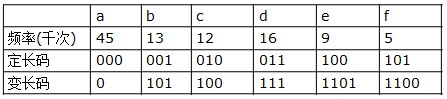有多种方式表示文件中的信息，若用0,1码表示字符的方法，即每个字符用唯一的一个0,1串表示。若采用定长编码表示，则需要3位表示一个字符，整个文件编码需要300,000位；若采用变长编码表示，给频率高的字符较短的编码；频率低的字符较长的编码，达到整体编码减少的目的，则整个文件编码需要（45×1+13×3+12×3+16×3+9×4+5×4）×1000=224,000位，由此可见，变长码比定长码方案好，总码长减小约25%。

前缀码：对每一个字符规定一个0,1串作为其代码，并要求任一字符的代码都不是其他字符代码的前缀。这种编码称为前缀码。编码的前缀性质可以使译码方法非常简单；例如001011101可以唯一的分解为0,0,101,1101，因而其译码为aabe。

译码过程需要方便的取出编码的前缀，因此需要表示前缀码的合适的数据结构。为此，可以用二叉树作为前缀码的数据结构：树叶表示给定字符；从树根到树叶的路径当作该字符的前缀码；代码中每一位的0或1分别作为指示某节点到左儿子或右儿子的“路标”。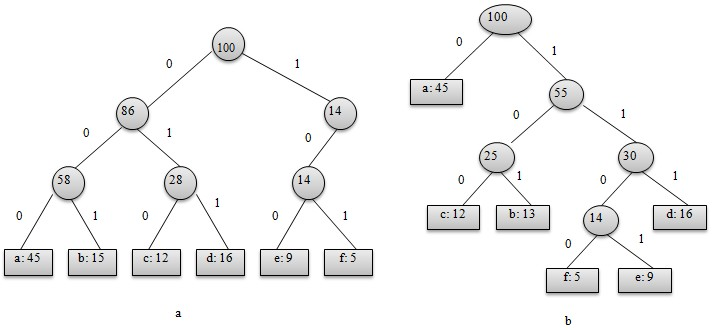从上图可以看出，表示最优前缀码的二叉树总是一棵完全二叉树，即树中任意节点都有2个儿子。图a表示定长编码方案不是最优的，其编码的二叉树不是一棵完全二叉树。在一般情况下，若C是编码字符集，表示其最优前缀码的二叉树中恰有|C|个叶子。每个叶子对应于字符集中的一个字符，该二叉树有|C|-1个内部节点。

给定编码字符集C及频率分布f,即C中任一字符c以频率f(c)在数据文件中出现。C的一个前缀码编码方案对应于一棵二叉树T。字符c在树T中的深度记为dT(c)。dT(c)也是字符c的前缀码长。则平均码长定义为：![这里写图片描述](https://img-blog.csdn.net/20151220143712799)使平均码长达到最小的前缀码编码方案称为C的最优前缀码。

2、构造哈弗曼编码

哈夫曼提出构造最优前缀码的贪心算法，由此产生的编码方案称为哈夫曼编码。其构造步骤如下：

(1)哈夫曼算法以自底向上的方式构造表示最优前缀码的二叉树T。

(2)算法以|C|个叶结点开始，执行|C|－1次的“合并”运算后产生最终所要求的树T。

(3)假设编码字符集中每一字符c的频率是f(c)。以f为键值的优先队列Q用在贪心选择时有效地确定算法当前要合并的2棵具有最小频率的树。一旦2棵具有最小频率的树合并后，产生一棵新的树，其频率为合并的2棵树的频率之和，并将新树插入优先队列Q。经过n－1次的合并后，优先队列中只剩下一棵树，即所要求的树T。

构造过程如图所示：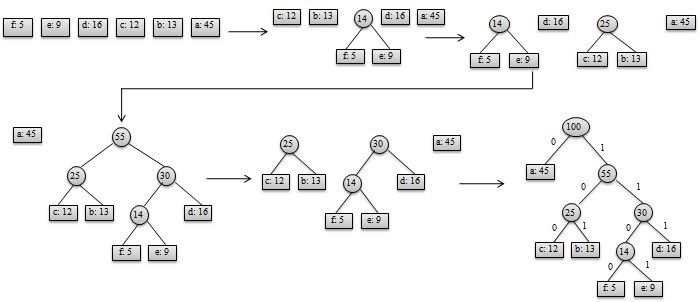/*-------------------------------------------------------------------------
* Name:   哈夫曼编码源
* Date:   2015.12.20
* Author: Ingrid
* 实现过程：
*      //初始化huffmanTree,huffmanCode
*      initHuffmanTree(huffmanTree,m);
*      initHuffmanCode(huffmanCode,n);
*
*      //获取huffmanCode的符号
*      getHuffmanCode(huffmanCode,n);
*
*      //获取huffmanTree的频数
*      getHuffmanWeight(huffmanTree,n);
*
*      //创建huffmanTree
*      createHaffmanTree(huffmanTree,n);
*      //创建huffmanCode
*      createHaffmanCode(huffmanTree,huffmanCode,n);
*
*      //输出huffmanCode编码
*      ouputHaffmanCode(huffmanCode,n);
*------------------------------------------------------------------------*/

import java.util.Scanner;
public class HuffmanCode{
//建立数的节点类
static class Node{
int weight;//频数
int parent;
int leftChild;
int rightChild;

public Node(int weight,int parent,int leftChild,int rightChild){
this.weight=weight;
this.parent=parent;
this.leftChild=leftChild;
this.rightChild=rightChild;
}

void setWeight(int weight){
this.weight=weight;
}

void setParent(int parent){
this.parent=parent;
}

void setLeftChild(int leftChild){
this.leftChild=leftChild;
}

void setRightChild(int rightChild){
this.rightChild=rightChild;
}

int getWeight(){
return weight;
}

int getParent(){
return parent;
}

int getLeftChild(){
return leftChild;
}

int getRightChild(){
return rightChild;
}
}

//新建哈夫曼编码
static class NodeCode{
String character;
String code;
NodeCode(String character,String code){
this.character=character;
this.code=code;
}
NodeCode(String code){
this.code= code;
}

void setCharacter(String character){
this.character=character;
}

void setCode(String code){
this.code=code;
}

String getCharacter(){
return character;
}

String getCode(){
return code;
}
}

//初始化一个huffuman树
public static void initHuffmanTree(Node[] huffmanTree,int m){
for(int i=0;i<m;i++){
huffmanTree[i] = new Node(0,-1,-1,-1);
}
}

//初始化一个huffmanCode
public static void initHuffmanCode(NodeCode[] huffmanCode,int n){
for(int i=0;i<n;i++){
huffmanCode[i]=new NodeCode("","");
}
}

//获取huffmanCode的符号
public static void getHuffmanCode(NodeCode[] huffmanCode , int n){
Scanner input = new Scanner(System.in);
for(int i=0;i<n;i++){
String temp = input.next();
huffmanCode[i] = new NodeCode(temp,"");
}
}

//获取huffman树节点频数
public static void getHuffmanWeight(Node[] huffmanTree , int n){
Scanner input = new Scanner(System.in);
for(int i=0;i<n;i++){
int temp = input.nextInt();
huffmanTree[i] = new Node(temp,-1,-1,-1);
}
}

//从n个结点中选取最小的两个结点
public static int[] selectMin(Node[] huffmanTree ,int n)
{
int min[] = new int;
class TempNode
{
int newWeight;//存储权
int place;//存储该结点所在的位置

TempNode(int newWeight,int place){
this.newWeight=newWeight;
this.place=place;
}

void setNewWeight(int newWeight){
this.newWeight=newWeight;
}

void setPlace(int place){
this.place=place;
}

int getNewWeight(){
return newWeight;
}

int getPlace(){
return place;
}
}

TempNode[] tempTree=new TempNode[n];

//将huffmanTree中没有双亲的结点存储到tempTree中
int i=0,j=0;
for(i=0;i<n;i++)
{
if(huffmanTree[i].getParent()==-1&& huffmanTree[i].getWeight()!=0)
{
tempTree[j]= new TempNode(huffmanTree[i].getWeight(),i);
j++;
}
}

int m1,m2;
m1=m2=0;
for(i=0;i<j;i++)
{
if(tempTree[i].getNewWeight()<tempTree[m1].getNewWeight())//此处不让取到相等，是因为结点中有相同权值的时候，m1取最前的
m1=i;
}
for(i=0;i<j;i++)
{
if(m1==m2)
m2++;//当m1在第一个位置的时候，m2向后移一位
if(tempTree[i].getNewWeight()<=tempTree[m2].getNewWeight()&& i!=m1)//此处取到相等，是让在结点中有相同的权值的时候，

//m2取最后的那个。
m2=i;
}

min=tempTree[m1].getPlace();
min=tempTree[m2].getPlace();
return min;
}

//创建huffmanTree
public static void createHaffmanTree(Node[] huffmanTree,int n){
if(n<=1)
System.out.println("Parameter Error!");
int m = 2*n-1;
//initHuffmanTree(huffmanTree,m);

for(int i=n;i<m;i++)
{
int[] min=selectMin(huffmanTree,i);
int min1=min;
int min2=min;
huffmanTree[min1].setParent(i);
huffmanTree[min2].setParent(i);
huffmanTree[i].setLeftChild(min1);
huffmanTree[i].setRightChild(min2);
huffmanTree[i].setWeight(huffmanTree[min1].getWeight()+ huffmanTree[min2].getWeight());
}
}

//创建huffmanCode
public static void createHaffmanCode(Node[] huffmanTree,NodeCode[] huffmanCode,int n){
Scanner input = new Scanner(System.in);
char[] code = new char;
int start;
int c;
int parent;
int temp;

code[n-1]='0';
for(int i=0;i<n;i++)
{
StringBuffer stringBuffer = new StringBuffer();
start=n-1;
c=i;
while( (parent=huffmanTree[c].getParent()) >=0 )
{
start--;
code[start]=((huffmanTree[parent].getLeftChild()==c)?'0':'1');
c=parent;

}
for(;start<n-1;start++){
stringBuffer.append(code[start]);
}
huffmanCode[i].setCode(stringBuffer.toString());
}
}

//输出hufmanCode
public static void ouputHaffmanCode(NodeCode[] huffmanCode,int n){
System.out.println("字符与编码的对应关系如下：");
for(int i=0;i<n;i++){
System.out.println(huffmanCode[i].getCharacter()+":"+huffmanCode[i].getCode());
}
}

//主函数
public static void main(String[] args){
Scanner input = new Scanner(System.in);
int n;
int m;
System.out.print("请输入字符个数：");
n = input.nextInt();
m=2*n-1;
Node[] huffmanTree = new Node[m];
NodeCode[] huffmanCode = new NodeCode[n];

//初始化huffmanTree,huffmanCode
initHuffmanTree(huffmanTree,m);
initHuffmanCode(huffmanCode,n);

//获取huffmanCode的符号
System.out.print("请输入哈夫曼编码的字符：");
getHuffmanCode(huffmanCode,n);

//获取huffmanTree的频数
System.out.print("请输入哈夫曼编码字符对应的频数：");
getHuffmanWeight(huffmanTree,n);

//创建huffmanTree
createHaffmanTree(huffmanTree,n);
//创建huffmanCode
createHaffmanCode(huffmanTree,huffmanCode,n);

//输出huffmanCode编码
ouputHaffmanCode(huffmanCode,n);

}
}

展开全文• 一、哈夫曼树的基本概念在一棵二叉树中，定义从A结点到B结点所经过的分支序列叫做从A结点到B结点的路径。从A结点到B结点所经过的分支个数叫做从A结点到B结点的路径长度从二叉树的根结点到二叉树中所有叶结点的路径...
一、哈夫曼树的基本概念
在一棵二叉树中，定义从A结点到B结点所经过的分支序列叫做从A结点到B结点的路径。从A结点到B结点所经过的分支个数叫做从A结点到B结点的路径长度
从二叉树的根结点到二叉树中所有叶结点的路径长度之和称作该二叉树的路径长度。

如果二叉树中的叶结点都带有权值，我们可以把这个定义加以推广。设二叉树有n个带权值的叶结点，定义从二叉树的根结点到二叉树中所有叶结点的路径长度与相应叶结点权值的乘积之和为该二叉树的带权路径长度（WPL），即：
WPL =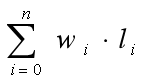wi为第i个叶结点的权值，li为从根结点到第i个叶结点的路径长度。
二、哈夫曼树
具有相同叶结点和不同带权路径长度的二叉树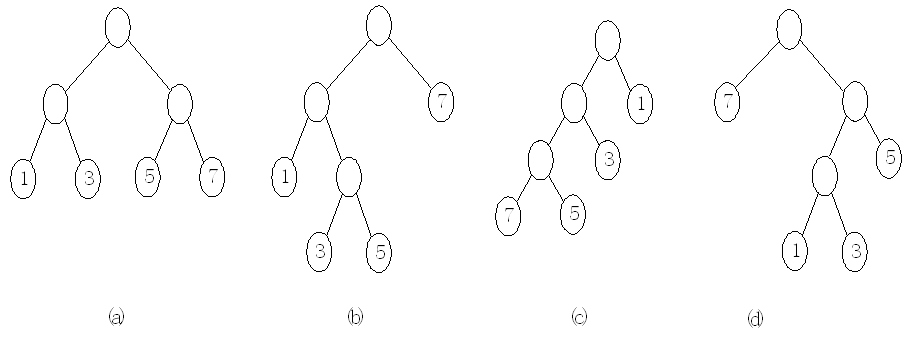（a）WPL = 1×2+3×2+5×2+7×2 = 32（b）WPL = 1×2+3×3+5×3+7×1 = 33（c）WPL = 7×3+5×3+3×2+1×1 = 43
（d）WPL = 1×3+3×3+5×2+7×1 = 29
三、哈夫曼树构造算法
根据哈夫曼树的定义，要使一棵二叉树的带权路径长度WPL值最小，必须使权值越大的叶结点越靠近根结点。哈夫曼提出的构造哈夫曼树构造算法为：（1）由给定的n个权值{w1,w2,…,wn}构造n棵只有根 结点的二叉树，从而得到一个二叉树森林F={T1,T2,…,Tn}。
（2）在二叉树森林F中选取根结点的权值最小和次小的两棵二叉树作为新的二叉树的左右子树构造新的二叉树，新的二叉树的根结点权值为左右子树根结点权值之和。
（3）在二叉树森林F中删除作为新二叉树左右子树的两棵二叉树，将新二叉树加入到二叉树森林F中。
（4）重复步骤（2）和（3），当二叉树森林F中只剩下一棵二叉树时，这棵二叉树就是所构造的哈夫曼树。
对于一组给定的叶结点，设它们的权值集合为{7,5,3,1}，按哈夫曼树构造算法对此集合构造哈夫曼树的过程如图所示。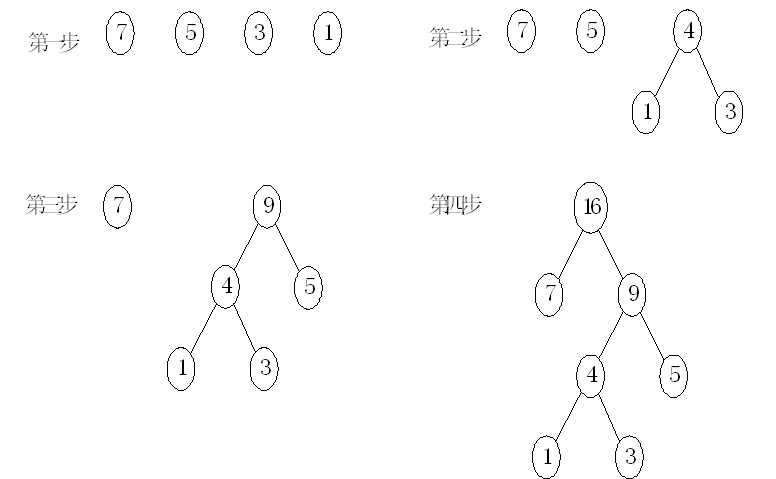四、哈夫曼树编码问题
哈夫曼树可用于构造代码总长度最短的编码方案。具体构造方法如下：
设需要编码的字符集合为{d1,d2,…,dn}，各个字符在电文中出现的次数集合为{w1,w2,…,wn}，以d1,d2,…,dn作为叶结点，以w1,w2,…,wn作为各叶结点的权值构造一棵二叉树，规定哈夫曼树中的左分支为0，右分支为1，则从根结点到每个叶结点所经过的分支对应的0和1组成的序列便为该结点对应字符的编码。这样的代码总长度最短的不等长编码称之为哈夫曼编码。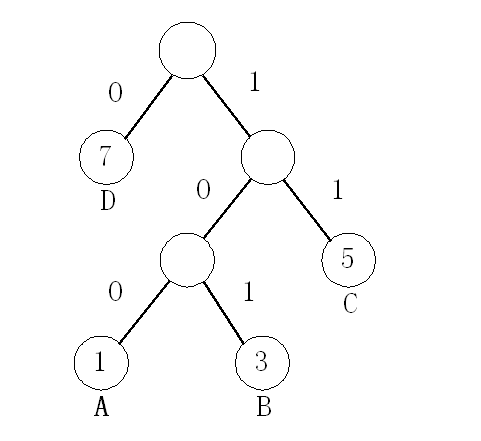哈夫曼编码的数据结构设计
设计哈夫曼树的结点存储结构为双亲孩子存储结构。存放哈夫曼编码的存储结构为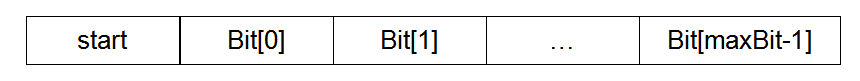五、哈夫曼树以及哈夫曼编码的实现
//哈夫曼树的结点类
public class HaffNode {

int weight; //权值
int parent;//双亲
int flag;//标志
int leftChild; //左孩子
int rightChild;//右孩子

HaffNode() {

}

}
//哈夫曼编码类
public class Code {

int[] bit;  //编码数组
int start;  //编码的开始下标
int weight; //权值

Code(int n) {
bit = new int[n];
start = n - 1;
}
}
/哈夫曼树类
public class HaffmanTree {
//最大权值
static final int MAXVALUE = 1000;
int nodeNum; //叶子结点个数

public HaffmanTree(int n) {
this.nodeNum = n;
}

//构造哈夫曼树算法
public void haffman(int[] weight, HaffNode[] nodes) {
int n = this.nodeNum;
//m1,m2,表示最小的两个权值，x1,x2,表示最小两个权值对应的编号
int m1, m2, x1, x2;

//初始化所有的结点，对应有n个叶子结点的哈夫曼树，有2n-1个结点。
for (int i = 0; i < 2 * n - 1; i++) {
HaffNode temp = new HaffNode();
//初始化n个叶子结点
if (i < n) {
temp.weight = weight[i];
} else {
temp.weight = 0;
}
temp.parent = 0;
temp.flag = 0;
temp.leftChild = -1;
temp.rightChild = -1;
nodes[i] = temp;
}

//初始化n-1个非叶子结点
for (int i = 0; i < n - 1; i++) {
m1 = m2 = MAXVALUE;
x1 = x2 = 0;
for (int j = 0; j < n + i; j++) {
if (nodes[j].weight < m1 && nodes[j].flag == 0) {
m2 = m1;
x2 = x1;
m1 = nodes[j].weight;
x1 = j;
} else if (nodes[j].weight < m2 && nodes[j].flag == 0) {
m2 = nodes[j].weight;
x2 = j;
}
}
nodes[x1].parent = n + i;
nodes[x2].parent = n + i;
nodes[x1].flag = 1;
nodes[x2].flag = 1;
nodes[n + i].weight = nodes[x1].weight + nodes[x2].weight;
nodes[n + i].leftChild = x1;
nodes[n + i].rightChild = x2;
}

}

//哈弗曼编码算法
public void haffmanCode(HaffNode[] nodes, Code[] haffCode) {
int n = this.nodeNum;
Code code = new Code(n);
int child, parent;

for (int i = 0; i < n; i++) {
code.start = n - 1;
code.weight = nodes[i].weight;
child = i;
parent = nodes[child].parent;
while (parent != 0) {
if (nodes[parent].leftChild == child) {
code.bit[code.start] = 0;
} else {
code.bit[code.start] = 1;
}

code.start--;
child = parent;
parent = nodes[child].parent;
}

Code temp = new Code(n);
for (int j = code.start + 1; j < n; j++) {
temp.bit[j] = code.bit[j];
}
temp.weight = code.weight;
temp.start = code.start;
haffCode[i] = temp;
}

}
}
public class Test {

public static void main(String[] args) {

int n = 4;
int[] weight = {1, 3, 5, 7};
HaffmanTree haffTree = new HaffmanTree(n);
HaffNode[] nodes = new HaffNode[2 * n - 1];
Code[] codes = new Code[n];
//构造哈夫曼树
haffTree.haffman(weight, nodes);
//生成哈夫曼编码
haffTree.haffmanCode(nodes, codes);

//打印哈夫曼编码
for (int i = 0; i < n; i++) {
System.out.print("Weight=" + codes[i].weight + " Code=");
for (int j = codes[i].start + 1; j < n; j++) {
System.out.print(codes[i].bit[j]);
}
System.out.println();
}
}

}
运行结果：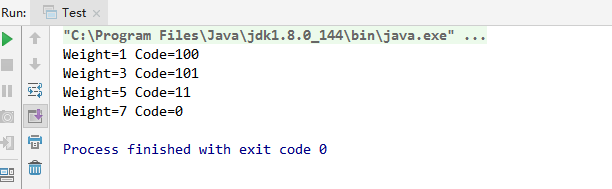展开全文• #include <stdlib.h>/*哈夫曼树建立、哈夫曼编码算法的实现*/ #include typedef char* HuffmanCode;/*动态分配数组，存储哈夫曼编码*/ typedef struct { unsigned int weight ; /* 用来存放各个结点的权值*/...input include 存储 struct
• 哈夫曼编码是一种变长的字符编码方式，常用于对指定的字符集进行数据压缩，压缩率在 20%～90%。1. 问题描述现在有一个包含 5 个字符的字符表 {A,B,C,D,E}，各字符出现的频率统计如表 1 所示。表 1：各字符出现的频率...
• ## 哈夫曼编码

千次阅读 2020-12-21 17:08:34
上一篇文章中提到数据结构：哈夫曼树，今天接着学习由哈夫曼提出编码方式，一种程序算法 简称：...哈夫曼（Huffman）编码算法 它是基于二叉树构建编码压缩结构的，它是数据压缩中经典的一种算法：根据文本字符出现的频补码 java
• 学校数据结构实验（c语言描述）布置了写求哈夫曼编码的作业，书上采用的是从叶子到根逆向求编码的方式（严蔚敏，清华大学出版社，数据结构c语言版），我这里给出先序遍历，即“根左右”进行哈夫曼编码的代码 ...哈弗曼树 c语言 先序遍历
• 本文主要针对输入的十个整型数，进行归一化之后，构建合适的哈夫曼树，在哈夫曼树的基础上进行哈夫曼编码设计，并就构造哈夫曼树和进行哈夫曼编码算法进行了较为细致的描述。本文另附二叉树的遍历搜索源码，较为...
• 本程序是VS2010下的源程序，可直接运行。...本程序实现了通过读取文件中关于字符的相关说明数据来初始化相关变量，最后采用贪心算法的思想编程实现哈夫曼编码的求解。最终输出各个字符的哈弗曼编码值。
• 0023 算法笔记贪心算法哈夫曼编码问题 1 问题描述 哈夫曼编码是广泛地用于数据文件压缩的十分有效的编码方法 其 压缩率通常在 20% 90% 之间哈夫曼编码 算法 用字符在文件中出现的 频率表来建立一个用 0 1 串表示各...
• 哈夫曼树1.1 基本概念算法思想贪心算法(以局部最优，谋求全局最优)适用范围1 【(约束)可行】：它必须满足问题的约束2 【局部最优】它是当前步骤中所有可行选择中最佳的局部选择3 【不可取消】选择一旦做出，在...
• 它用C语言详细地介绍了哈弗曼编码的贪心算法的设计步骤及数据描述
• 0023算法笔记贪心算法哈夫曼编码问题 ?1问题描述 ? ? ?哈夫曼编码是广泛地用于数据文件压缩十分有效编码方法其压缩率通常在20%90%之间哈夫曼编码 \o "算法和数据结构知识库" 算法用字符在文件中出现频率表来建立一个...
• 哈夫曼编码 简介 哈夫曼编码是一种字符编码方式，可以对指定的字符集进行数据压缩，压缩率在20%到90%。 问题描述 现在有一个包含5个字符{A,B,C,D,E}，各个字符的出现频率依次为{0.35, 0.1, 0.2, 0.2, 0.15}。...
• 运用哈夫曼算法构造哈夫曼树，并得到哈夫曼编码。 输入格式：10,5,21,18,8,13 二、实验目的 掌握哈夫曼算法。 三、实验内容及要求 1、构造哈夫曼树和哈夫曼编码的存储结构。 2、实现哈夫曼算法，实现哈夫曼树的存储...
• 0023 算法笔记贪心算法哈夫曼编码问题 1问题描述 哈夫曼编码是广泛地用于数据文件压缩的十分有效的编码方法 其压缩率通常在 20%90%之间哈夫曼编码算法用字符在文件中出 现的频率表来建立一个用 01 串表示各字符的...
• 1、C语言 - 哈夫曼编码实验报告福建工程学院课程设计课程：数据结构题目：哈夫曼编码和译码专业：信息管理信息系统班级：1002 班座号：15 号姓名：林左权2011 年6 月27 日2实验题目：哈夫曼编码和译码一、要解决的...
• 1、C语言 - 哈夫曼编码实验报告福建工程学院课程设计课程：数据结构题目：哈夫曼编码和译码专业：信息管理信息系统班级：1002 班座号：15 号姓名：林左权2011 年6 月27 日2实验题目：哈夫曼编码和译码一、要解决的...
• 文件名称：第十一周项目1 - 哈夫曼编码算法验证.cpp 作 者：孟琪琪 完成日期：2016年11月10日 版 本 号：v1.0 问题描述: 运行并重复测试教学内容中涉及的算法。改变测试数据进行重复测试的意义在于， 可以...
• 1、C语言-哈夫曼编码实验报告11课程:题目:专业:班级:座号:姓名:福建工程学院课程设计数据结构 哈夫曼编码和译码 信息管理信息系统1002 班 15号 林左权2011年 6月 27日实验题目：哈夫曼编码和译码一、要解决的问题...
• 哈夫曼编码算法用字符在文件中出现的频率表来建立一个用0，1串表示各字符的最优表示方式。一个包含100,000个字符的文件，各字符出现频率不同，设某信源产生a，b，c，d和f 6种符号，其频率见下表,单位为千次。 从表...二叉树 数据结构
• 与哈夫曼树一样，会不会有小伙伴对哈夫曼编码很陌生，有疑惑问题疑惑1.哈夫曼编码是做什么的？有什么用？2.为什么要使用哈夫曼编码？使用别的编码行不行？哈夫曼编码是做什么的？有什么用？哈夫曼(Huffm...补码 字符串 python java...